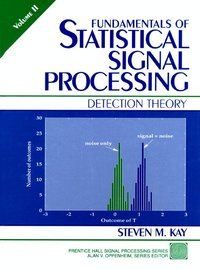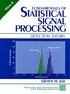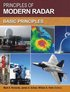Fler böcker inom
Format
Inbunden (Hardback)
Språk
Engelska
Antal sidor
576
Utgivningsdatum
1998-01-01
Upplaga
1
Förlag
Pearson
Illustratör/Fotograf
Illustrations
Illustrationer
Illustrations
Volymtitel
Detection Theory
Dimensioner
240 x 185 x 30 mm
Vikt
1020 g
Antal komponenter
1
Komponenter
2 v. :
ISBN
9780135041352

### Du kanske gillar# Fundamentals of Statistical Signal Processing, Volume II

Detection Theory

Inbunden Engelska, 1998-01-01
1236
Skickas inom 5-8 vardagar.
Fri frakt inom Sverige för privatpersoner.
The most comprehensive overview of signal detection available.

This is a thorough, up-to-date introduction to optimizing detection algorithms for implementation on digital computers. It focuses extensively on real-world signal processing applications, including state-of-the-art speech and communications technology as well as traditional sonar/radar systems.

Start with a quick review of the fundamental issues associated with mathematical detection, as well as the most important probability density functions and their properties. Next, review Gaussian, Chi-Squared, F, Rayleigh, and Rician PDFs, quadratic forms of Gaussian random variables, asymptotic Gaussian PDFs, and Monte Carlo Performance Evaluations.

Three chapters introduce the basics of detection based on simple hypothesis testing, including the Neyman-Pearson Theorem, handling irrelevant data, Bayes Risk, multiple hypothesis testing, and both deterministic and random signals.

The author then presents exceptionally detailed coverage of composite hypothesis testing to accommodate unknown signal and noise parameters. These chapters will be especially useful for those building detectors that must work with real, physical data. Other topics covered include:

• Detection in nonGaussian noise, including nonGaussian noise characteristics, known deterministic signals, and deterministic signals with unknown parameters
• Detection of model changes, including maneuver detection and time-varying PSD detection
• Complex extensions, vector generalization, and array processing
The book makes extensive use of MATLAB, and program listings are included wherever appropriate. Designed for practicing electrical engineers, researchers, and advanced students, it is an ideal complement to Steven M. Kay's Fundamentals of Statistical Signal Processing, Vol. 1: Estimation Theory (Prentice Hall PTR, 1993, ISBN: 0-13-345711-7).

## Passar bra ihop

1.2. +
3.De som köpt den här boken har ofta också köpt Principles of Modern Radar: Volume 1 av Mark A Richards, James A Scheer, William A Holm (inbunden).

## Kundrecensioner

Har du läst boken? Sätt ditt betyg »

## Övrig information

STEVEN M. KAY is Professor of Electrical Engineering at the University of Rhode Island and a leading expert in signal processing.

## Innehållsförteckning

(NOTE: Most chapters begin with an Introduction and Summary.)

1. Introduction.

Detection Theory in Signal Processing. The Detection Problem. The Mathematical Detection Problem. Hierarchy of Detection Problems. Role of Asymptotics. Some Notes to the Reader.

2. Summary of Important PDFs.

Fundamental Probability Density Functionshfil Penalty - M and Properties. Quadratic Forms of Gaussian Random Variables. Asymptotic Gaussian PDF. Monte Carlo Performance Evaluation. Number of Required Monte Carlo Trials. Normal Probability Paper. MATLAB Program to Compute Gaussian Right-Tail Probability and its Inverse. MATLAB Program to Compute Central and Noncentral c 2 Right-Tail Probability. MATLAB Program for Monte Carlo Computer Simulation.

3. Statistical Decision Theory I.

Neyman-Pearson Theorem. Receiver Operating Characteristics. Irrelevant Data. Minimum Probability of Error. Bayes Risk. Multiple Hypothesis Testing. Neyman-Pearson Theorem. Minimum Bayes Risk Detector - Binary Hypothesis. Minimum Bayes Risk Detector - Multiple Hypotheses.

4. Deterministic Signals.

Matched Filters. Generalized Matched Filters. Multiple Signals. Linear Model. Signal Processing Examples. Reduced Form of the Linear Model1.

5. Random Signals.

Estimator-Correlator. Linear Model1. Estimator-Correlator for Large Data Records. General Gaussian Detection. Signal Processing Example. Detection Performance of the Estimator-Correlator.

6. Statistical Decision Theory II.

Composite Hypothesis Testing. Composite Hypothesis Testing Approaches. Performance of GLRT for Large Data Records. Equivalent Large Data Records Tests. Locally Most Powerful Detectors. Multiple Hypothesis Testing. Asymptotically Equivalent Tests - No Nuisance Parameters. Asymptotically Equivalent Tests - Nuisance Parameters. Asymptotic PDF of GLRT. Asymptotic Detection Performance of LMP Test. Alternate Derivation of Locally Most Powerful Test. Derivation of Generalized ML Rule.

7. Deterministic Signals with Unknown Parameters.

Signal Modeling and Detection Performance. Unknown Amplitude. Unknown Arrival Time. Sinusoidal Detection. Classical Linear Model. Signal Processing Examples. Asymptotic Performance of the Energy Detector. Derivation of GLRT for Classical Linear Model.

8. Random Signals with Unknown Parameters.

Incompletely Known Signal Covariance. Large Data Record Approximations. Weak Signal Detection. Signal Processing Example. Derivation of PDF for Periodic Gaussian Random Process.

9. Unknown Noise Parameters.

General Considerations. White Gaussian Noise. Colored WSS Gaussian Noise. Signal Processing Example. Derivation of GLRT for Classical Linear Model for s 2 Unknown. Rao Test for General Linear Model with Unknown Noise Paramet...